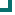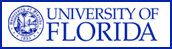Enterococcus faecium (NATIONAL Data) 2004 2005 2006 2007 2008 2009 2010 ampicillin 12.7%n=1216 10.9%n=1185 10.7%n=2051 12.8%n=1966 12.3%n=1827 11.3%n=573 31.9%n=72 vancomycin 41.2%n=1910 40.9%n=1579 28.7%n=2506 27.8%n=2210 27.7%n=1983 28.8%n=656 47.2%n=72 quinupristin/dalfopristin 84.9%n=205 74.6%n=67 92.9%n=238 56.5%n=262 98.6%n=70 97.8%n=89 nitrofurantoin 56.5%n=741 48.5%n=549 24.4%n=1398 10.7%n=1134 11.4%n=1031 13.1%n=360 tetracycline 38%n=790 51.6%n=579 57.1%n=1199 57.7%n=1144 62.7%n=1192 48.4%n=279 45.5%n=11 gentamicin 72%n=460 77.1%n=70 84.6%n=208 83.7%n=98 10.2%n=157 amikacin 7.7%n=26 9.1%n=11 chloramphenicol 95.2%n=397 98.6%n=73 98.3%n=705 100%n=37 100%n=33 rifampim 30.2%n=86 42.4%n=33 10.2%n=49 21.9%n=64 13.3%n=30 16.1%n=62 28.6%n=28 Totals 44.3%n=5805 36.8%n=4161 36.6%n=8354 27.9%n=6915 27.9%n=6323 26.3%n=2030 38.3%n=183

This data is accurate as of: 11/22/2016 11:53:15 AM

Drugs with less than 10 isolates are removed from the chart and from the graph. The following drugs had some data removed: rifampim, quinupristin/dalfopristin, gentamicin, amikacin, chloramphenicol, ampicillin, vancomycin, tetracycline.ARMProgram.com is a project run by the University of Florida. Copyright 2011. All Rights Reserved. Site by JMarc Technologies, LLC